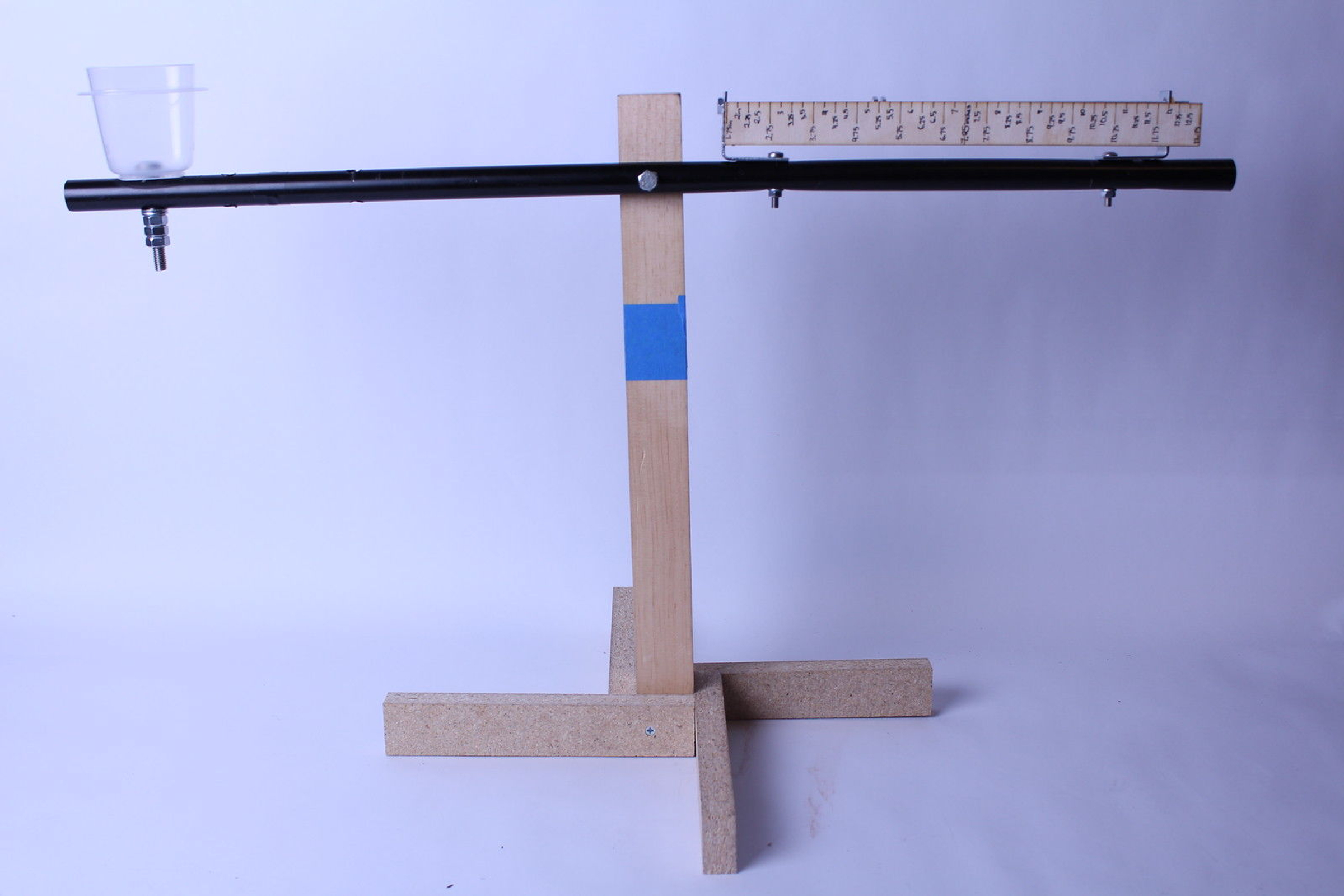# Final## Final

Shivani Angappan1 / 6

This precision balance is made entirely of commonly-found material, and was designed and built without the use of any CAD software. It is a torque balance, operated as follows:

First, one places the mass one wishes to measure in the plastic holder on the left side of the balance. Then, the nuts on the threaded rod on the right side of the balance are moved along the rod, to the right if the non-calibrated mass is too heavy, and to the left if the non-calibrated mass is too light. Then, the radial distance of the nuts is found by averaging all the distances of the nuts from the fulcrum (this can be read straight off the attached ruler). Then, one performs the math below in order to obtain the mass of the non-calibrated object.

Here's the math necessary to work the balance.

First off, let's call the mass on the left side of the plank, the mass that we want to measure, m_n, for non-calibrated mass. Then, the force of gravity on this mass is F_n = m_n * g, so the torque of this object relative to the fulcrum of the balance is T_n = F_n * r_n, where r_n is the radial distance relative to the fulcrum. We know that r_n is a fixed constant, because we want the non-calibrated mass to be right in the center of the left side of the plank. We can say that the plank has length R, so r_n  = 0.5R. Then, we know that T_n = F_n * 0.5R = m_n * g * 0.5R.

Now, let's look at the side with the calibrated mass. The way I designed the balance is for it to have movable nuts on a threaded rod, so the total torque on the calibrated mass side is the average of the torque of each separate nut, so if we let the mass of each nut be m_i and the radial distance of each nut be r_i (where 0 < i <= total number of nuts), then the total torque on the calibrated mass side is g * (∑_[i = 1]^[number of nuts] {m_i * r_i})/(number of nuts) = T_c.

In order for the calibrated mass and the non calibrated mass to be perfectly equal, we need to have the torques be equal. Thus, we need to equate our expression for T_c and T_n.

Now, we have that T_c = T_n, so g * (∑_[i = 1]^[number of nuts] {m_i * r_i})/(number of nuts) = m_n * g * 0.5R.

All of the quantities in that newest equation are defined except for m_n, so we solve for that.

Thus, we have that m_n = (g * (∑_[i = 1]^[number of nuts] {m_i * r_i})/(number of nuts)) / (0.5 R).

So, to operate the balance just right so that the masses are balanced, we need to adjust the cart on its slider along the track so that its radial distance relative to the fulcrum makes the equation come out just right. Thus, all someone would need to do to operate the balance is move the slider until the two sides of the plank are perfectly balanced (to the naked eye, which means that they will only be *very close* to perfectly balanced), and then solve the equation for m_n.